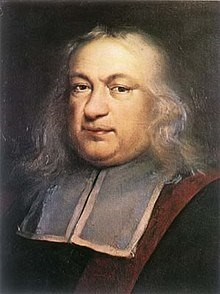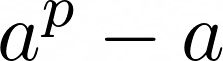## FermatPierre de Fermat (1607-1665) was a French lawyer at the Parlement of Toulouse, France, and a mathematician who is given credit for early developments that led to infinitesimal calculus, including his technique of adequality. In particular, he is recognized for his discovery of an original method of finding the greatest and the smallest ordinates of curved lines, which is analogous to that of differential calculus, then unknown, and his research into number theory. He made notable contributions to analytic geometry, probability, and optics. He is best known for Fermat’s principle for light propagation and his Last Theorem in number theory,

Fermat’s little theorem states that if p is a prime number, then for any integer a, the numberis an integer multiple of p. In the notation of modular arithmetic, this is expressed asFor example, if a = 2 and p = 7, then 2⁷= 128, and 128 – 2 = 126 = 7 × 18 is indeed an integer multiple of 7.

If a is not divisible by p, Fermat’s little theorem is equivalent to the statement thatis an integer multiple of p, or in symbols:For example, if a = 2 and p = 7, then 2⁶ = 64, and 64 – 1 = 63 = 7 × 9 is indeed a multiple of 7.

Fermat’s Last Theorem states that no three positive integers a, b, and c satisfy the equationfor any integer value of n greater than 2. The cases n = 1 and n = 2 have been known since antiquity to have infinitely many solutions.

In a note at the margin of a copy of Diophantus’ Arithmetica, he stated “I have discovered a truly marvelous proof of this, which this margin is too narrow to contain.” That was a bold, unsubstantiated claim. It wasn’t until 1995 that Andrew Wiles succeeded in proving the theorem.